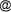## Alvaro Cañadas, Frantiscek Vilcko: Tree Volume and Taper Function for Cordia alliodora in Agroforestry System, Protected Forest Sumaco

ALVARO CAÑADAS, FRANTISCEK VILCKO
Georg-August-Universität Göttingen, Institute of Forest Management, Germany

The Protected Forest Sumaco is characterised by extensive areas of secondary forests. It provides a buffer zone for the National Park Sumaco-Napo-Galeras at an altitude of 1,160m above sea level and with a precipitation of 4,000 mm year-1. Adequate management of secondary forest in this protected area requires basic data about growth and volume- dependent regeneration of Laurel in agroforestry systems. Consequently, accurate and flexible methods are required to estimate stand and tree growth and yield. Since the introduction of regression methods into forestry, it has been common practice to select models for growth and yield based on diagnostic and model selection criteria, and to estimate equation coefficients for specific data. Mathematical properties, such as algebraic consistency, have been applied to improve model specification. A system of equations is called algebraically (also referred to as mathematically) consistent if one equation in the system can be expressed in terms of the other equation(s). For example, if an individual tree volume equation can be expressed in terms of a taper function, or vice versa, the system of equations is algebraically consistent. The objective of this study was to derive a system of equations in order to determine the volume and taper function that is both, mathematically and numerically, consistent for Cordia alliodora. For the parameterisation of the total volume and taper function 77 trees were taken. Altogether 308 observations were used, such as DBH, the diameter at 2, 4, 6, 8 meters and the total height. The volume was estimated with the Smalian Formula for five sections. The two models used in this research were validated, using 44 independent trees from the neighbouring community of Wawa Sumaco.

Keywords: Dimensional analysis, Ecuador, Laurel, local management system, mathematical consistent

#### Footnotes

...PContact Address: Alvaro Cañadas, Georg-August-Universität Göttingen, Institute of Forest ManagementBüsgenweg 5, 37077 Göttingen, Germany, e-mail: acanadauni-forst.gwdg.de
Andreas Deininger, September 2004